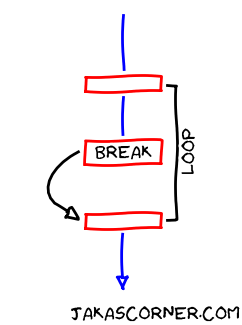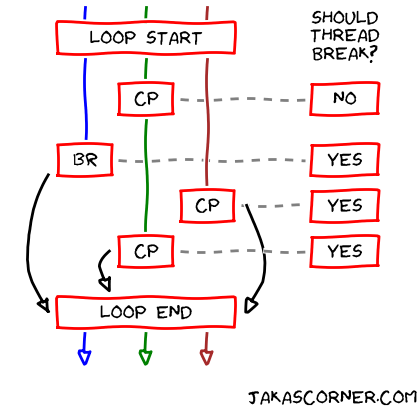In a sequential program, we can exit a loop with the break statement.But it gets more complicated when dealing with parallel programs. Here are some difficulties which comes to my mind:

How should threads behave in a parallel program with “the break statement”? What should a thread do when it reaches the break?

I suppose the thread should exit the loop when it reaches the break statement.

But what should then the other threads do? Should they also exit the loop at the same time as the thread which encountered the break? Or should the other threads continue with the execution as if nothing happened?

Neither of these options sound as a good idea…

The break statement is a simple feature in sequential programs. But it is not trivial to do it in parallel programs. However, there are some options.

OpenMP provides us mechanisms which can mimic the break statement in parallel programs.

## Exiting parallel loops early

Let us assume that we have a parallel loop with a “parallel break” statement. Now, we describe how OpenMP ends the parallel loop early if a thread reaches the “parallel break”.

When a thread reaches the “parallel break”, OpenMP signals to the other threads that someone just reached the “parallel break”. The thread (which encountered the break) then exits the parallel loop.

How does OpenMP signal to the other threads? Well, it does not signal them directly. It writes to an internal variable which indicates that the “parallel break” was reached.

How do the other threads receive the signal? They check the internal variable. If the variable says that the “parallel break” was reached, then the other threads also finish their execution of the loop.

The important question is: When does a thread check the internal variable?

OpenMP defines special locations in the program called the cancellation points. When a thread reaches a cancellation point, it checks the internal variable.

Let us examine the described mechanism with an artificial example.

## Example

The following figure presents the outline of the example.There are three threads: the blue, the green and the brown thread. The threads parallelize the loop with the break statement.

The green thread first encounters `CP`. Abbreviation `CP` stands for the cancellation point.

At the cancellation point, the green thread checks the internal variable. We represent the internal variable on the right side with the question “Should thread break?”. Since the internal variable says that the thread should not exit the loop (the answer is `NO`), the green thread continues executing the loop.

Then, the blue thread reaches `BR`, which stands for the break statement.

Two things happen at this point. OpenMP sets the internal variable to `true`, which means that the other threads should now exit the loop when they encounter a cancellation point. The figure describes this on the right side, where the answer to “Should thread break?” is set to `YES`.

Afterwards, the blue thread exits the loop.

Now, the brown thread reaches `CP`. The thread checks the internal variable and breaks out of the loop.

Finally, the green thread reaches its second `CP`. Similar as in the case of the brown thread, the green thread checks the internal variable and exits the loop.

## Summary

In this article, we described the OpenMP model for exiting the loops. The model assumes that a thread sends a cancellation signal to the other threads. The other threads then check for the signal at the so called cancellation points. If a thread at a cancellation point receives the signal, the thread exits the loop.

In the next article, we will explain how to implement the cancellation of the loops with the OpenMP library.